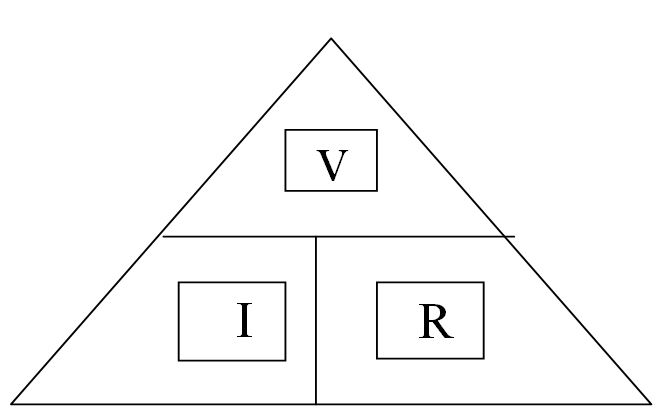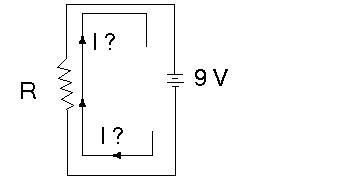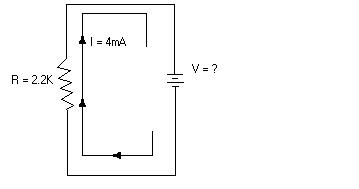#### Ohm's Law

Ohm's law states that (provided that the temperature of an electric conductor does not change) the current going through it is proportional to the potential difference (i.e. Voltage) across it.
''Proportional'' means that doubling the voltage doubles the current halving the voltage, halves the current etc.
From this law we may arrive at a very imported equation in the field of electronics:

## V= I x R

Where V is the voltage measured in volts, I is the current measured in amperes and R is the resistance measured in ohms (W)
Which may also be written as, I = V/R And R = V/I

You can use the following triangle to help you remember all three forms of this important equationUsing the equation   V= I * R, solve the following problems:

Question l:
A filament lamp passes a current of 60 mA (0. 06 A) when the voltage is 6V What is the resistance of the filament?

Answer
R=V/I
= 6V/ 0.06 A
= 100 ohms ( 100 W)

Question 2:
What is the amount of current flowing through a 30 ohm fixed value resistor when it is connected to a 9V battery?
Schematic diagramSolution:

##### I = V/R
= (9 V / 30 W)

= 0.3 A
= 300 mA

Question 3:

What is the voltage across the 2.2 kW(2200W) in the circuit below?Solution:
V= I x R
= 2.2kW x 4 mA

= 2200W x 0.004A

= 8.8 V